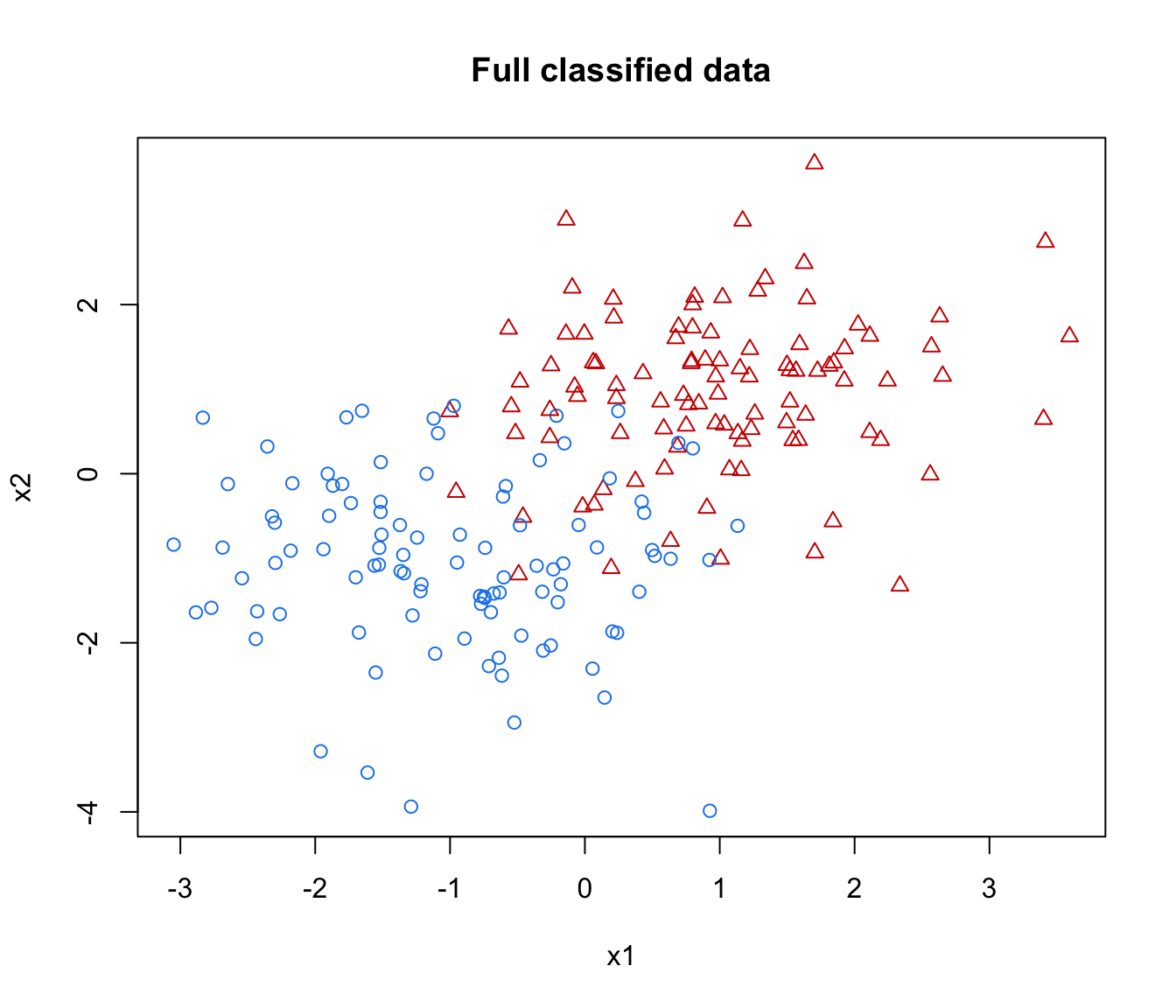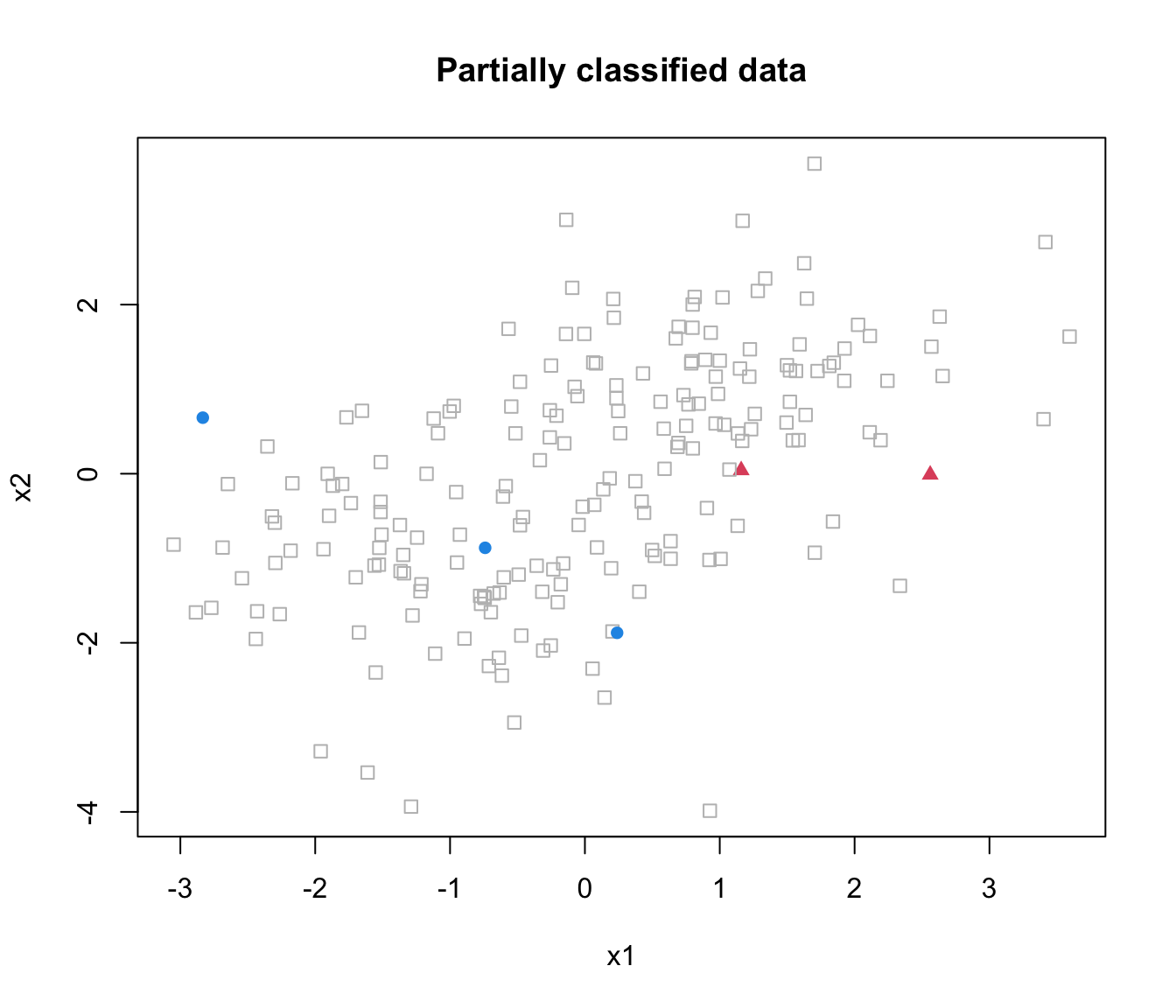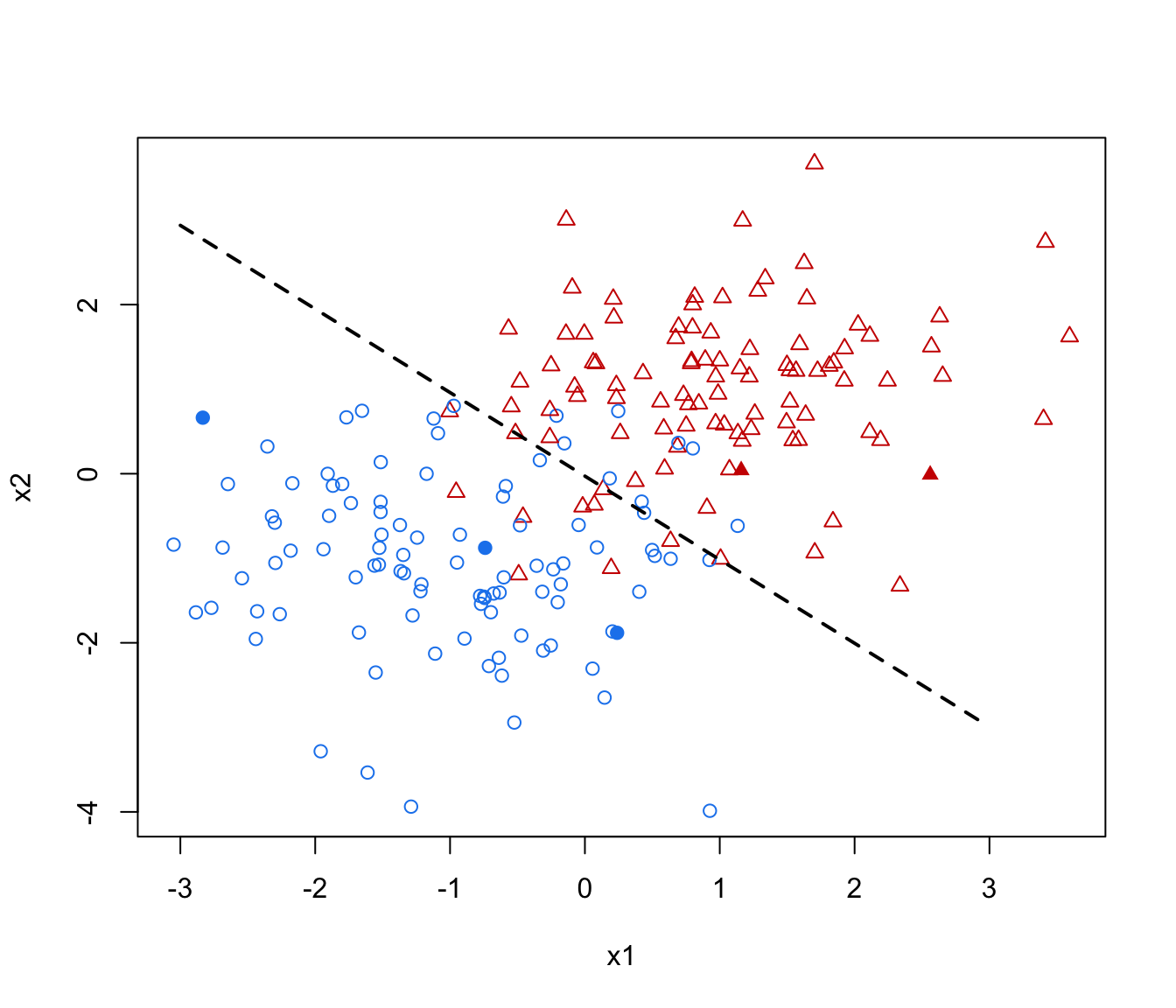Semi-Supervised classification based on Gaussian finite mixture modeling.

MclustSSC(data, class,
G = NULL, modelNames = NULL,
prior = NULL, control = emControl(),
warn = mclust.options("warn"),
verbose = interactive(),
...)

## Arguments

data

A data frame or matrix giving the training data.

class

A vector giving the known class labels (either a numerical value or a character string) for the observations in the training data. Observations with unknown class are encoded as NA.

G

An integer value specifying the numbers of mixture components or classes. By default is set equal to the number of known classes. See the examples below.

modelNames

A vector of character strings indicating the models to be fitted by EM (see the description in mclustModelNames). See the examples below.

prior

The default assumes no prior, but this argument allows specification of a conjugate prior on the means and variances through the function priorControl.

control

A list of control parameters for EM. The defaults are set by the call emControl().

warn

A logical value indicating whether or not certain warnings (usually related to singularity) should be issued when estimation fails. The default is controlled by mclust.options.

verbose

A logical controlling if a text progress bar is displayed during the fitting procedure. By default is TRUE if the session is interactive, and FALSE otherwise.

...

Further arguments passed to or from other methods.

## Value

An object of class 'MclustSSC' providing the optimal (according to BIC) Gaussian mixture model for semi-supervised classification. The details of the output components are as follows:

call

The matched call.

data

The input data matrix.

class

The input class labels (including NAs for unknown labels.

modelName

A character string specifying the "best" estimated model.

G

A numerical value specifying the number of mixture components or classes of the "best" estimated model.

n

The total number of observations in the data.

d

The dimension of the data.

BIC

All BIC values.

loglik

Log-likelihood for the selected model.

df

Number of estimated parameters.

bic

Optimal BIC value.

parameters

A list with the following components:

pro

A vector whose kth component is the mixing proportion for the kth component of the mixture model.

mean

The mean for each component. If there is more than one component, this is a matrix whose kth column is the mean of the kth component of the mixture model.

variance

A list of variance parameters for the model. The components of this list depend on the model specification. See the help file for mclustVariance for details.

z

A matrix whose [i,k]th entry is the probability that observation i in the test data belongs to the kth class.

classification

The classification corresponding to z, i.e. map(z).

prior

The prior used (if any).

control

A list of control parameters used in the EM algorithm.

## Details

The semi-supervised approach implemented in MclustSSC() is a simple Gaussian mixture model for classification where at the first M-step only observations with known class labels are used for parameters estimation. Then, a standard EM algorithm is used for updating the probabiltiy of class membership for unlabelled data while keeping fixed the probabilities for labelled data.

## Author

Luca Scrucca

summary.MclustSSC, plot.MclustSSC, predict.MclustSSC

## Examples

# Simulate two overlapping groups
n <- 200
pars <- list(pro = c(0.5, 0.5),
mean = matrix(c(-1,1), nrow = 2, ncol = 2, byrow = TRUE),
variance = mclustVariance("EII", d = 2, G = 2))
pars$variance$sigmasq <- 1
data <- sim("EII", parameters = pars, n = n, seed = 12)
class <- data[,1]
X <- data[,-1]
clPairs(X, class, symbols = c(1,2), main = "Full classified data")# Randomly remove labels
cl <- class; cl[sample(1:n, size = 195)] <- NA
table(cl, useNA = "ifany")
#> cl
#>    1    2 <NA>
#>    3    2  195
clPairs(X, ifelse(is.na(cl), 0, class),
symbols = c(0, 16, 17), colors = c("grey", 4, 2),
main = "Partially classified data")# Fit semi-supervised classification model
mod_SSC  <- MclustSSC(X, cl)
summary(mod_SSC, parameters = TRUE)
#> ----------------------------------------------------------------
#> Gaussian finite mixture model for semi-supervised classification
#> ----------------------------------------------------------------
#>
#>  log-likelihood   n df       BIC
#>       -654.5776 200  6 -1340.945
#>
#> Classes   n    % Model G
#>    1      3  1.5   EII 1
#>    2      2  1.0   EII 1
#>    <NA> 195 97.5
#>
#> Mixing probabilities:
#>         1         2
#> 0.5022706 0.4977294
#>
#> Means:
#>            1         2
#> x1 -1.002123 0.9946794
#> x2 -1.038680 0.9812348
#>
#> Variances:
#> 1
#>          x1       x2
#> x1 0.912807 0.000000
#> x2 0.000000 0.912807
#> 2
#>          x1       x2
#> x1 0.912807 0.000000
#> x2 0.000000 0.912807
#>
#> Classification summary:
#>       Predicted
#> Class   1  2
#>   1     3  0
#>   2     0  2
#>   <NA> 99 96

pred_SSC <- predict(mod_SSC)
table(Predicted = pred_SSC$classification, Actual = class) #> Actual #> Predicted 1 2 #> 1 91 10 #> 2 9 90 ngrid <- 50 xgrid <- seq(-3, 3, length.out = ngrid) ygrid <- seq(-4, 4.5, length.out = ngrid) xygrid <- expand.grid(xgrid, ygrid) pred_SSC <- predict(mod_SSC, newdata = xygrid) col <- mclust.options("classPlotColors")[class] pch <- class pch[!is.na(cl)] = ifelse(cl[!is.na(cl)] == 1, 19, 17) plot(X, pch = pch, col = col) contour(xgrid, ygrid, matrix(pred_SSC$z[,1], ngrid, ngrid),
add = TRUE, levels = 0.5, drawlabels = FALSE, lty = 2, lwd = 2)